##### Geometry: 1001 Practice Problems For Dummies (+ Free Online Practice)Geometric series are relatively simple but important series that you can use as benchmarks when determining the convergence or divergence of more complicated series. A geometric series is a series of the form: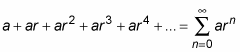The first term, a, is called the leading term. Each term after the first equals the preceding term multiplied by r, which is called the common ratio.

For example, if a is 5 and r is 3, you get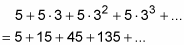You just multiply each term by 3 to get the next term. By the way, the 3 in this example is called the common ratio because the ratio of any term divided by its preceding term equals 3, but it probably makes a lot more sense to think of the 3 as your multiplier.

If a is 100 and r is 0.1, you get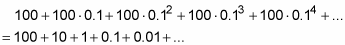And if a is 1/2 and r is also 1/2, you get the series: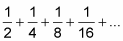The convergence/divergence rule for geometric series is a snap.

Geometric Series Rule: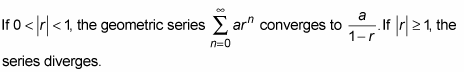(Note that this rule works when –1 < r < 0, in which case you get an alternating series.)

In the first example, a = 5 and r = 3, so the series diverges. In the second example, a is 100 and r is 0.1, so the series converges to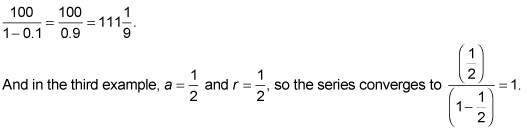This is how far you walk if you start 1 yard from the wall, then step half way to the wall, then half of the remaining distance, and so on and so on. You take an infinite number of steps, but travel a mere yard. And how long will it take you to get to the wall? Well, if you keep up a constant speed and don’t pause between steps (which of course is impossible), you’ll get there in the same amount of time it would take you to walk any-old yard. If you do pause between steps, even for a billionth of a second, you’ll never get to the wall.